The "Two-Dimensional Factorial"
and a New Solution for the Probability
of Completing Sets in Random Sampling

Jeffrey D. Lindsay

(This is a Web adaptation of a paper that I published while I was an Associate Professor at the Institute of Paper Science and Technology in Atlanta, Georgia. Original reference: J.D. Lindsay, "A New Solution for the Probability of Completing Sets in Random Sampling: Definition of the 'Two-Dimensional Factorial'," The Mathematical Scientist, 17: 101-110 (1992).)

# Abstract

In developing an improved solution to a classical sampling problem, a new recursive function is obtained which can be termed a "two-dimensional factorial." The sampling problem deals with the probability of completing a subset of unique items when randomly sampled with replacement from a finite population. The two-dimensional factorial is partially tabulated, and several of its properties are investigated, including limits for large numbers. Use of this function offers significant computational advantages over the previous classical solution to the probability problem considered here. The function is not known to have been discovered in previous work.

# Introduction

A classical sampling problem in probability theory deals with the likelihood of collecting a set of items by randomly sampling a population (Feller, 1950, pp. 51-66). A simple example can be found in the collection of sets of promotional items offered inside cereal boxes or other consumable goods. The items are presumably randomly and uniformly distributed, and remain unidentified until the package has been opened. For instance, the makers of one cereal brand offered miniature license plates from all 50 states with one plate per box. If I want to collect all 50, how many boxes should I plan to purchase to be 95% confident that the set will be completed? A less ambitious consumer may simply want to know the probability that at least 10 different plates will be obtained by purchasing 12 boxes. Related questions may be found in sampling problems in scientific studies.

We will begin by considering the simple problem when the different classes in the population each compose an equal fraction of the population. In general terms, our problem statement becomes:

If U unique classes of items are randomly and uniformly distributed among an infinite population, what is the probability that a specified number, U – M, of the unique classes will be acquired in N trials? (M is the number of missing classes in the sample.)

We will introduce the notation P(N,U,M) to denote this probability. Feller (1950, pp. 64, 69) shows that this probability is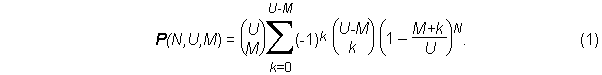By taking an independent approach in the solution, we will show a new form for the solution to be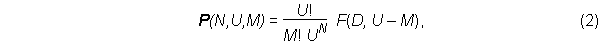where D is the number of duplicate items among the N samples, or D = N -(U - M), and F is a recursive function defined byAfter derivation of the probability formulas, we will discuss properties and limiting values of the recursive function F, which can be described as a two-dimensional factorial function.

# Derivation of the Two-dimensional Factorial

The two-dimensional factorial function was found by examining patterns in calculating the permutations for obtaining U – M unique items in N trials. The number of such permutations divided by the total number of possible permutations, UN, gives the desired probability. For example, consider the problem of collecting all three items of a set in six tries. Here U = 3, M = 0, and N = 6, and the number of duplicates, D, is 3. The permutations are treated in Table 1. There are 10 cases to consider, one for each feasible combination of positions occupied by the duplicates. Duplicates are shown in bold type. For example, in case 6, duplicates occur at trials 2, 5, and 6. For trial 1, any of the three unique items can be chosen. If a duplicate occurs in trial 2, there is only one possibility, the same item that was selected in the first trial. The remaining two items appear in trials 3 and 4, so the number of possibilities becomes 2 and 1, respectively. For trials 5 and 6, any selection will be a duplicate, so the number of possibilities becomes 3 and 3.

Table 1. Permutations for the 10 possible cases when U=3, N = 6, and M = 0.

 Case Trials Permutations 1. 3 1 1 1 2 1 =3!x(1x1x1) 2. 3 1 1 2 2 1 =3!x(1x1x2) 3. 3 1 1 2 1 3 =3!x(1x1x3) 4. 3 1 2 2 2 1 =3!x(1x2x2) 5. 3 1 2 2 1 3 =3!x(1x2x3) 6. 3 1 2 1 3 3 =3!x(1x3x3) 7. 3 2 2 2 2 1 =3!x(2x2x2) 8. 3 2 2 2 1 3 =3!x(2x2x3) 9. 3 2 2 1 3 3 =3!x(2x3x3) 10. 3 2 1 3 3 3 =3!x(3x3x3) Total  =  3!x90  =  3!xF(3,3)

The total number of permutations is the product of 3! and the total permutations for duplicates, which is the sum of the products in parentheses in the rightmost column of Table 1. The sum of numbers in parentheses can be written as eitheror as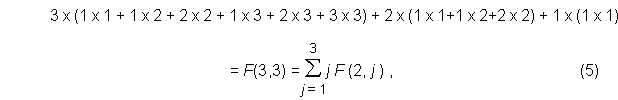whereThe number of cases is given by the number of feasible combination of positions for the D duplicates among N = U – M + D sample positions, with duplicates able to occur only after at least one element of U has been selected. The number of cases is thus (N–1)!/(D!(N – D – 1)!). The number of choices available for a duplicate equals the number of unique items previously selected in that case.

In general, when all U distinct items are selected in an arbitrary N >= U trials, there are U! permutations for the first selections of these items. For the D = N – U duplicates, the kth duplicate can be any one of ak items, where ak denotes the number of distinct items already selected, 1 <= a1 <= a2 ... <= aD <= U. The number of permutations of duplicates is then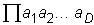. Summing over all possible values of aD, the number of permutations for obtaining all U distinct items in N trials, resulting in D = N – U duplicates, is therefore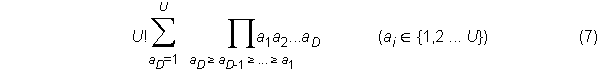which can also be written as U! F(D,U), where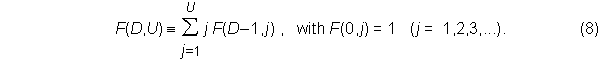When M of the U unique items are missing in the sampled subset, the number of duplicates becomes D = N (U – M). By considering the permutations of duplicates and first occurrences, as was done in deriving equation (7), it is easily shown that the total number of permutations becomes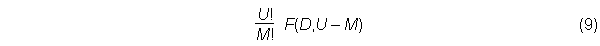with the function F the same as defined in equation (8). In general, then, the probability of obtaining U – M unique items from a possible U items, distributed uniformly throughout an infinite population, in N trials is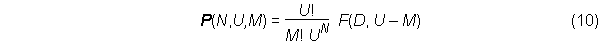where D = N (U – M) is the number of duplicate items among the N samples and F is a recursive function defined by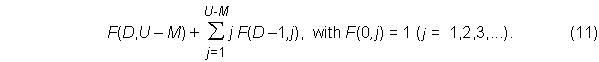Equating the right-hand sides of equations (1) and (10) and simplifying yields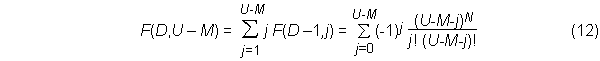The identity in equation (12) is by no means obvious and is an interesting result of itself.

The probability P(N,U,M) can be computed using either equation (10) or equation (1) from Feller. Likewise, F(D,U – M) can be determined using the recursive approach of equation (11), or the alternating-sign series in equation (12). Use of the recursive function offers a significant computational advantage, for it is a summation of positive terms only, whereas the alternating-sign series involves small differences of large numbers. Limited numerical resolution on a computer thus greatly restricts the usefulness of equation (1). For example, to compute F(D= 4, U = 43, M = 0) = 8.04E+11 with the alternating-sign series, differences between numbers 16 orders of magnitude greater are required. From j = 6 to j = 11, the terms of the series are 1.45E+27, -2.04E+27, 2.35E+27, -2.25E+27, 1.80E+27, and -1.22E+27. Summing the series on a computer with 15 digits of resolution (the Wingz™ spreadsheet by Informix was used on a Macintosh II) yielded a negative result, whereas accuracy was maintained with the recursive approach until sums exceeded the largest allowed number, 1.7E+308.

# Further Properties of the Two-dimensional Factorial

The two-dimensional factorial appears to be an interesting function meriting further study. Table 2 shows values of F(D,U – M) for 1 <= D <= 25 and 1 <= U – M <= 7. Several interesting features are apparent in the columns of numbers shown here. Note that F(D,1) = 1 and F(D,2) = 2D+1 - 1 for all D. A logarithmic contour plot in Figure 1 for the range 1 <= D <= 30 and 1 <= U – M <= 29 shows how the numbers increase with U and D.

Table 2.  F(D,U – M) for 1 <= D <= 25 and 1 <= U – M <= 7.

 U - M 1 2 3 4 5 6 7 8 9 1 1 3 6 10 15 21 28 36 45 2 1 7 25 65 140 266 462 750 1 155 3 1 15 90 350 1 050 2 646 5 880 11 880 22 275 4 1 31 301 1 701 6 951 22 827 63 987 159 027 359 502 5 1 63 966 7 770 42 525 179 487 627 396 1 899 612 5 135 130 6 1 127 3 025 34 105 246 730 1 323 652 5 715 424 20 912 320 67 128 490 7 1 255 9 330 145 750 1 379 400 9 321 312 49 329 280 216 627 840 820 784 250 8 1 511 28 501 611 501 7 508 501 63 436 373 408 741 333 2 141 764 053 9 528 822 303 9 1 1 023 86 526 2 532 530 40 075 035 420 693 273 3 281 882 604 20 415 995 028 1.062E+11 10 1 2 047 261 625 10 391 745 210 766 920 2 734 926 558 25 708 104 786 1.890E+11 1.145E+12 11 1 4 095 788 970 42 355 950 1 096 190 550 17 505 749 898 1.975E+11 1.710E+12 1.201E+13 12 1 8 191 2 375 101 171 798 901 5 652 751 651 110 687 251 039 1.493E+12 1.517E+13 1.233E+14 D 13 1 16 383 7 141 686 694 337 290 28 958 095 545 693 081 601 779 1.114E+13 1.325E+14 1.242E+15 14 1 32 767 21 457 825 2 798 806 985 147 589 284 710 4.306E+12 8.231E+13 1.142E+15 1.232E+16 15 1 65 535 64 439 010 11 259 666 950 7.492E+11 2.659E+13 6.028E+14 9.742E+15 1.206E+17 16 1 131 071 193 448 101 45 232 115 901 3.791E+12 1.633E+14 4.383E+15 8.232E+16 1.168E+18 17 1 262 143 580 606 446 1.815E+11 1.914E+13 9.990E+14 3.168E+16 6.902E+17 1.120E+19 18 1 524 287 1 742 343 625 7.278E+11 9.642E+13 6.090E+15 2.278E+17 5.750E+18 1.066E+20 19 1 1 048 575 5 228 079 450 2.916E+12 4.850E+14 3.703E+16 1.632E+18 4.763E+19 1.007E+21 20 1 2 097 151 15 686 335 501 1.168E+13 2.437E+15 2.246E+17 1.165E+19 3.927E+20 9.453E+21 21 1 4 194 303 47 063 200 806 4.677E+13 1.223E+16 1.360E+18 8.289E+19 3.224E+21 8.830E+22 22 1 8 388 607 1.412E+11 1.872E+14 6.134E+16 8.220E+18 5.885E+20 2.638E+22 8.211E+23 23 1 16 777 215 4.236E+11 7.493E+14 3.074E+17 4.963E+19 4.169E+21 2.152E+23 7.605E+24 24 1 33 554 431 1.271E+12 2.999E+15 1.540E+18 2.993E+20 2.948E+22 1.751E+24 7.020E+25 25 1 67 108 863 3.813E+12 1.200E+16 7.713E+18 1.804E+21 2.082E+23 1.422E+25 6.460E+26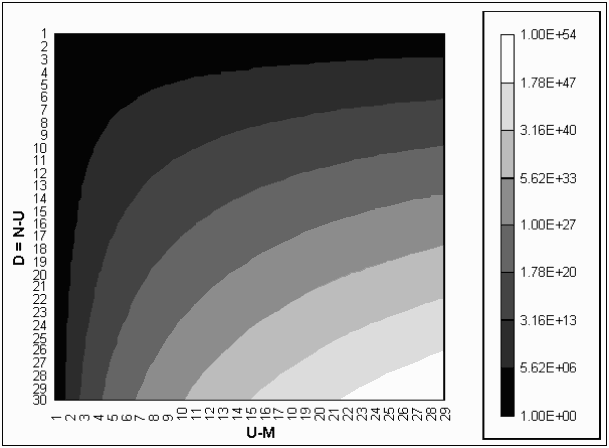Figure 1. Logarithmic contour plot of F(D,U – M) for 1 <= D <= 30 and 1 <= U – M <= 29.

## Limits for Large Numbers

As D, and hence N, becomes very large for a given U, P(N,U,0) approaches unity (it becomes nearly certain that all U items will be collected if enough samples are obtained). Thus,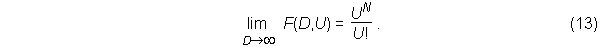Therefore, the ratio F(D,U)/F(D-1,U) approaches U for large D. Likewise, for large D, the ratio of adjacent values in any row is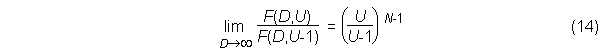A more exact expression than equation (13) is possible using a theorem for the limit of Equation (1) proved by Feller ((1950), pp. 72-75) and attributed by him (with a different proof) to von Mises (1939):

If U and N increase so that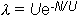remains bounded, then for fixed M: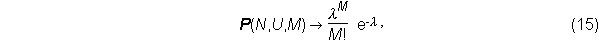which is the Poisson distribution. The two-dimensional factorial for large N = U + D is then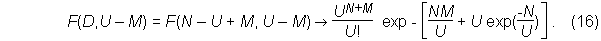For M = 0, this can be rewritten as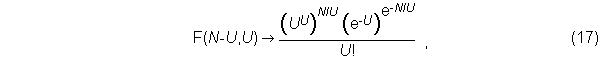or for finite M, we can re-express Equation (16) as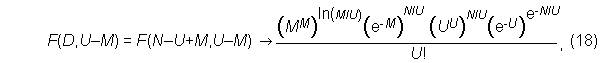where the terms in the numerator bear some resemblance to Stirling's formula for large factorials,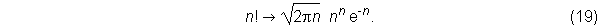While the resemblance to the regular factorial function is somewhat superficial, the two-dimensional factorial is still suggested as an appropriate name for the recursive F function introduced here. The main similarity to the factorial is through the recursive expression given in equation (11).

Comparisons of the approximate form in equation (15) with the exact probability form in equation (10) suggest that the approximate form must be used with caution for M>0. For example, for a given U and M, the approximation may be close for a certain range of N, but will become increasingly incorrect as N increases.

## Number Analysis

One feature of the numbers produced by the two-dimensional factorial is that a large proportion of them seem to have seven and eleven as factors. In Table 3 (a subset of Table 2), numbers divisible by seven are in italics, and numbers divisible by eleven are in bold. About 20% of the numbers examined are divisible by both seven and eleven. I have no explanation for this feature.

Table 3. Subset of Table 2 showing F(D, U – M) values divisible by seven in italic and values divisible by eleven are in boldface.

 U – M 1 2 3 4 5 6 7 1 1 3 6 10 15 21 28 2 1 7 25 65 140 266 462 3 1 15 90 350 1 050 2 646 5 880 4 1 31 301 1 701 6 951 22 827 63 987 5 1 63 966 7 770 42 525 179 487 627 396 6 1 127 3 025 34 105 246 730 1 323 652 5 715 424 7 1 255 9 330 145 750 1 379 400 9 321 312 49 329 280 8 1 511 28 501 611 501 7 508 501 63 436 373 408 741 333 9 1 1 023 86 526 2 532 530 40 075 035 420 693 273 3 281 882 604

Examination of the last digits of the numbers in columns 2 - 5 shows interesting repeating patterns if we consider that the initial, undisplayed row for D = 0 consists of 1s. The repeating final digits are:

Column 2: 1-3-7-5
Column 3: 1-6-5-0
Column 4: 1-0-5-0
Column 5: 1-5-0-0

Column 6 shows an interesting pattern in the final digits. The sequence is

1-1 –  6-6 – 7-7 – 2-2 – 3-3 – 8-8 – 9-9 – 4-4 – 5-5 – 0-0,

which apparently repeats (I am not sure because of limited numerical resolution). These pairs of digits change according to a specific pattern: add 5, add 1, subtract 5, add 1, and repeat.

# Applications and Examples

## Probability of Collecting at Least U – M Sets

P(N,U,M) in equation (10) gives the probability of obtaining exactly U – M identical sets in a random sample of size N from a uniform, infinite population. The collector, however, is usually more interested in the probability of collecting at least a specified number of distinct items. For distinct values of M the events "M items are missing" are disjoint. Therefore, the probability that no more than Mmax classes are missing in a random sample of size N is given by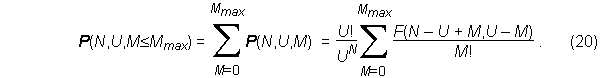Since the probability of having at least one distinct item is unity,## Expected Number of Trials to Complete a Set

For a series of U distinct items sampled with replacement, Feller (1950, pp. 174-175) shows that the expected number of samples to obtain U – M distinct items is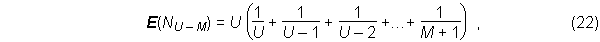which, for large U, can be approximated by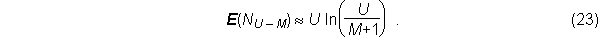In the limit of large U, E(N) = U ln(U) when M = 0. Applying the Poisson approximation to P(N,U,0) for large N, we see that the probability of collecting all U sets in E(N) trials approaches e-1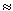0.3679 as U becomes large.

## Sample Probability Results

Table 4 shows the smallest number of samples required to complete a set of U distinct items (M = 0) with minimum p-values from 0.5 to 0.99.  Equation (10) was used for all values of U; for comparison, several results from the Poisson approximation in equation (15) are also shown in the last four rows. Based on Table 4, the would-be collector of items hidden in packages should plan on buying three to five times as many packages as there are items to be collected to be fairly sure (about 90% confident) of collecting a complete set with less than 20 items. For larger sets (more than 25 items, say), it may be necessary to buy six or more times as many packages as there are items to be collected.

For the case of M = 0, the Poisson approximation corresponds well to the exact results of equation (10). We noted above that the accuracy decreases substantially when M > 0. For examples, the probability of getting exactly 48 out of 50 distinct items (M = 2) from 159 samples is 0.297 from equation (10), but the Poisson approximation gives 0.270. However, cases with M >0 are often of less interest than completed sets.

In computing results for U = 25 and 50 in Table 4, rescaling F values was necessary to avoid numeric overflow. To rescale on a spreadsheet with columns of U and rows of D, divide a row of F values at constant D by a large number such as 10250 (the choice depends on the numerical limits of the computer and software and the value of U being considered). F values in subsequent rows (higher D) are then computed recursively with equation (8). To obtain P(N,U,M) from equation (1) using the reduced F values, replace UN in the denominator with the appropriately scaled number, e.g.,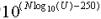, which may prevent the numeric overflow that can occur in evaluating UN. In rescaling, numeric underflow could occur in columns of small U, but these have a negligible effect on F at the larger U values where rescaling is needed.

Table 4. Samples required to complete sets of distinct items at several p-values.

 Smallest N required for a p-value of at least: 0.5 0.75 0.9 0.95 0.99 2 2 3 5 6 8 3 5 7 9 11 15 4 7 10 13 16 21 5 10 14 18 21 28 6 13 18 23 27 36 7 17 22 28 33 43 8 20 26 33 38 51 U 9 23 30 38 44 58 10 28 35 44 51 66 11 31 39 50 57 74 12 35 44 55 63 82 … … … … … … 25 90 111 135 152 192 … … … … … … 50 214 257 306 341 422 Estimates using equation (15): 5 10 15 20 23 32 12 35 45 57 66 86 U 25 90 112 137 155 196 50 214 258 308 345 426

For the case originally considered, U = 50 license plates, Table 5 shows the probabilities of partially completed sets with various M values if one buys N = 100 boxes. The likelihood of collecting plates from all 50 states is 0.00017, and the chance that no more than three states will be missing is only 5.18%. The most likely outcome is that six states will be missing, although there is a 52% probability that even more than six will be missing. With N = 180, the probability of completing the set is still only 24.5% (25.5% according to the approximation of equation (15)). To be 90% confident of getting all 50 states, one should have to purchase 308 boxes, as shown in Table 4. (Consumers may do well to simply contact the manufacturer of the collectable items and buy a complete set directly.)

Table 5. Probabilities for the case of U = 50 and N =100.

 M P(100,50,M) Cumulative 0 0.00017 0.00017 1 0.00202 0.00219 2 0.01129 0.01348 3 0.03835 0.05183 4 0.08910 0.14093 5 0.15071 0.29164 6 0.19294 0.48458 7 0.19183 0.67641 8 0.15082 0.82723 9 0.09501 0.92224 10 0.04841 0.97065 11 0.02009 0.99074 12 0.00682 0.99756 13 0.00190 0.99947 14 0.00044 0.99990 15 0.00008 0.99999

# Closure

A new form of the solution to a classical probability problem has yielded an interesting function which may be termed a two-dimensional factorial. The function allows computation of set collection probabilities with improved accuracy compared to the classical alternating-sign series solution in equation (1) for uniformly distributed populations.

# Acknowledgment

The author is grateful for the valuable comments and suggestions offered by Dr. Bruce Collings of the Brigham Young University Statistics Department and by Kendra L. Lindsay.

# References

Feller, W. (1950) An Introduction to Probability Theory and Its Applications, Vol. 1, John Wiley and Sons, New York.

von Mises, R. (1939) "Über Aufteilungs- und Besetzungswarhscheinlichkeiten," Revue de la Faculté des Sciences de l'Université d'Istanbul, N.S., 4 , 1-19, as cited by Feller, p. 72.

Curator: Jeff Lindsay,   Contact:
Last Updated: Jan. 17, 2001

URL: "http://www.jefflindsay.com/2dfactorial.shtml"
Site index